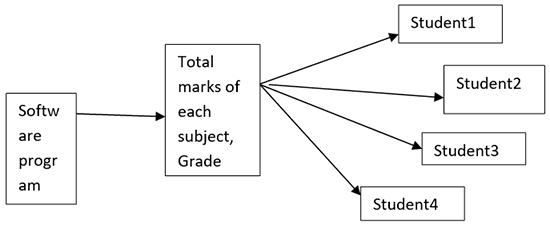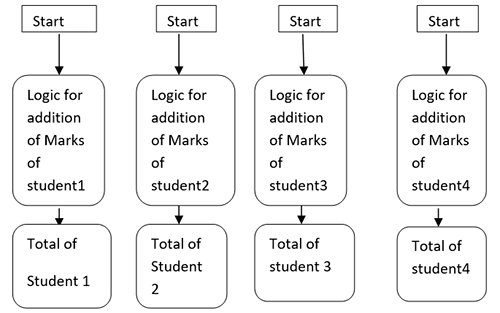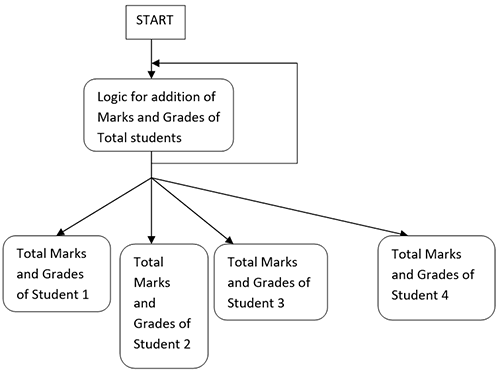Loop Requirement in Python | Loop | How works Loop in Python# Loop Requirement in Python

3321

What are the requirements of the loop in python?
Suppose you want to calculate the addition of marks and grades of all the subjects of every student in the class, for that you need to develop one python software.For developing the module, there is a need to print marks of every student in each subject and it total also. There are two methods for performing this task i.e. either manually or through looping.Manual method
Firstly, there is a need to write the logic for calculating the total of marks and grades of student and then you have to write that logic for all the students in the class. There might be more than 50 students in the class and for all 50 students, you need to repeatedly write the code. This process can be a very time-consuming process, hectic, complex and increase the size of the code of the program.

This problem is overcome by another method
i.e. looping. In which you have to write logic of calculating addition only once and keep on iterating that logic for total no students. When this loop run once, it will print the total  marks of the first student and when the loop run for the second time, it will print the total marks of the second student similarly it will keep on repeating. If we have 60 students, it will repeat for 60 times. Through looping, you do not need to write the logic for all the students. With one Logic, you can perform the task of 60 students. This will reduce the complexity and size of the code and increase the speed.

Flowchart of loopShare:## Get a .COM for just \$6.98

Secure Domain for a Mini Price